Normal view

# Linear models in statistics / Alvin C. Rencher and G. Bruce Schaalje.

Material type:TextLanguage: English Publisher: Hoboken, N.J. : Wiley-Interscience, c2008Edition: 2nd edDescription: xvi, 672 p. : ill. ; 25 cmISBN: 9780471754985 (cloth); 0471754986 (cloth)DDC classification: 519.535 LOC classification: QA276 | .R425 2008
Contents:
Table of contents Matrix algebra -- Random vectors and matrices -- Multivariate normal distribution -- Distribution of quadratic forms in y -- Simple linear regression -- Multiple regression : estimation -- Multiple regression : tests of hypotheses and confidence intervals -- Multiple regression : model validation and diagnostics -- Multiple regression : random x's -- Multiple regression : Bayesian inference -- Analysis-of-variance models -- One-way analysis-of-variance : balanced case -- Two-way analysis-of-variance : balanced case -- Analysis-of-variance : the cell mean model for unbalanced data -- Analysis-of-covariance -- Linear mixed models -- Additional models.
Summary: Summary: This new edition discusses classical linear models from a matrix algebra perspective, making the subject easily accessible to readers encountering linear models for the first time. It provides a Read more...
Tags from this library: No tags from this library for this title.Average rating: 0.0 (0 votes)
Item type Current location Collection Call number Copy number Status Date due Barcode Item holdsE-Book
E-book
Non-fiction 519.535 REL 2008 (Browse shelf) Not for loanText
Reserve Section
Non-fiction 519.535 REL 2008 (Browse shelf) C-1 Not For Loan 26926
Total holds: 0
##### Browsing EWU Library shelves, Shelving location: Reserve Section Close shelf browser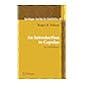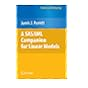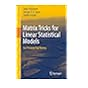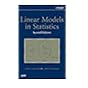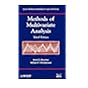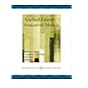519.535 NEI 2006 An introduction to copulas / 519.535 PES 2010 A SAS/IML companion for linear models / 519.535 PUM 2011 Matrix tricks for linear statistical models : 519.535 REL 2008 Linear models in statistics / 519.535 REM 2012 Methods of multivariate analysis / 519.536 ANR 2010 Regression with linear predictors / 519.536 APP 2005 Applied linear statistical models /

Includes bibliographical references and index.

Table of contents Matrix algebra --
Random vectors and matrices --
Multivariate normal distribution --
Distribution of quadratic forms in y --
Simple linear regression --
Multiple regression : estimation --
Multiple regression : tests of hypotheses and confidence intervals --
Multiple regression : model validation and diagnostics --
Multiple regression : random x's --
Multiple regression : Bayesian inference --
Analysis-of-variance models --
One-way analysis-of-variance : balanced case --
Two-way analysis-of-variance : balanced case --
Analysis-of-variance : the cell mean model for unbalanced data --
Analysis-of-covariance --
Linear mixed models --
Additional models.

Summary:
This new edition discusses classical linear models from a matrix algebra perspective, making the subject easily accessible to readers encountering linear models for the first time. It provides a Read more...

Applied Statistics

There are no comments for this item.

to post a comment.
Share

Library Home | Contacts | E-journals
Copyright @ 2011-2019 EWU Library
East West University